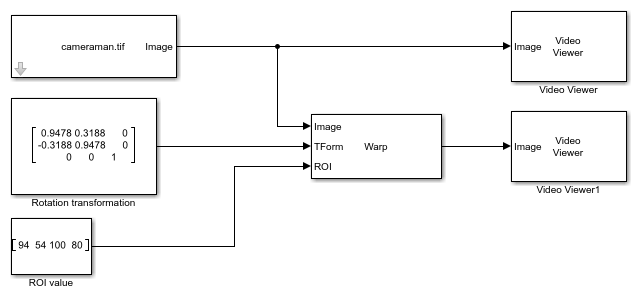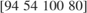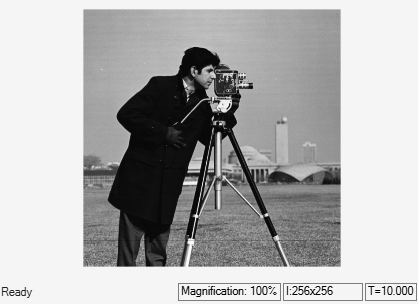Rotate ROI in Image

This example shows how to apply rotation transformation to a region of interest (ROI) in the input image.

Example Model

modelname = 'ex_rotatewarp.slx';
open_system(modelname)The model reads an input image by using the Image From File block. To apply rotation transformation to a particular region in the input image, you must

• specify the rotation transformation matrix

• specify the ROI

The model then rotates the selected ROI by using the Warp block with these parameter values:

• Transformation matrix source - Input port. This enables the TForm input port. Specify the rotation transformation matrix value asby using the Constant block.

• Interpolation method - Bilinear

• Background fill value - 0

• Output image position source - Same as input image

• Select the Enable ROI input port parameter. Specify the value for ROI input port asby using the Constant block.

Simulate and Display Results

Run the model and display the results by using the Video Viewer block.

sim(modelname);